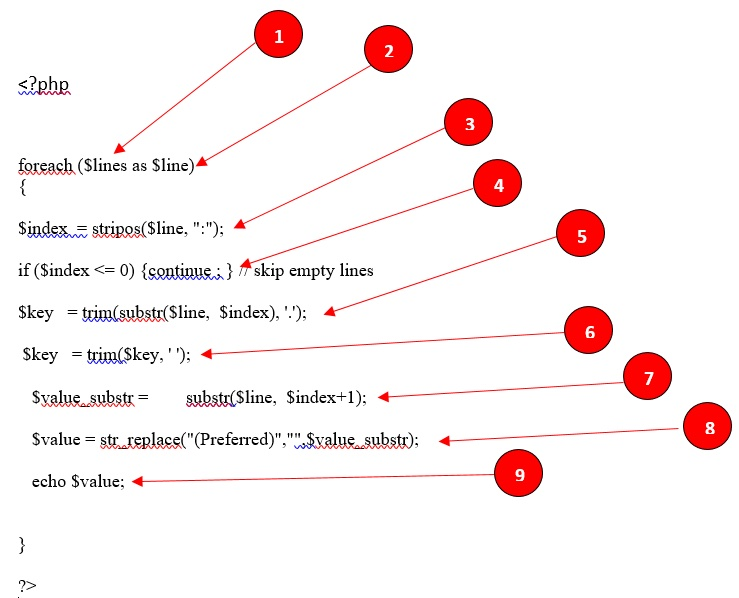# How to parse ipconfig data

Learn how to parse ipconfig results data.

You can parse ipconfig results data using PHP.The full code is:

``````<?php
foreach (Slines as Sline)
{
\$index = stripos(Sline, ":");
if (\$index <= 0) {continue;} // skips empty lines
\$key = trim(substr(\$line, \$index), '.');
\$key = trim(\$key,'');
\$value_substr = substr(\$line, \$index+1);
\$value = str_replace("(Preferred)", "", \$value_substr);
echo \$value;
}
?>``````

Here is the explanation of this code snippet:

1. The `\$lines` variable contains all the lines of ipconfig data in a form of string.
2. The foreach loop will pass every line one by one into the `\$line` variable.
3. The `\$index” variable will contain the index of`:`` in one line.
4. It would skip and continue the loop from start if the index is "0" (empty line).
5. It would fetch the loop key in each line by trimming the "." From the substring till the ":" index.
6. It would trim white spaces from the substring.
7. It would get the substring from every line after the ":" index.
8. It would reemove the "(Preferred)" word from the substring of each line after the ":" index if (Preferred) exists.